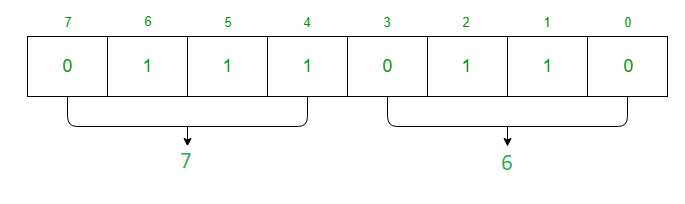# Interface 8254 PIT with 8085 microprocessor

• Last Updated : 23 Aug, 2018

Prerequisite – 8254 Control Register and Operating modes
Problem – Write an assembly language program in 8085 microprocessor which generates 1 KHz square waveform by using counter 1 as a binary counter if clock frequency of 8254 is 2 MHz.

Assumption – Assume the port addresses are 80 H, 81 H, 82 H, 83 H for C0(Counter 0), C1(Counter 1), C2(Counter 2), CR(Control Register).

Attention reader! Don’t stop learning now. Get hold of all the important CS Theory concepts for SDE interviews with the CS Theory Course at a student-friendly price and become industry ready.

For the above problem, 8254 must work in Mode 3 which is the square wave generator.
Count for register is given as clock frequency / square wave frequency
count = 2 MHz / 1 KHz
= 2000
= (07D0) H
Now the data is 16 bit so value of RW1 = 1 and RW0 = 1 in Control Register. As we want to select C1 (Counter 1) the value of SC1 = 0 and SC0 = 1 in Control Register. Value of M2 = 0, M1 = 1 and M2 = 1 for Mode 3 in Control Register. For binary counter value of LSB in CR is 0.

Hence the Control Register(CR) is given by,Algorithm –

1. Move the data 76 in A
2. Display the contents of A to port 83
3. Move the data D0 in A
4. Display the contents of A to port 81
5. Move the data 07 in A
6. Display the contents of A to port 81
7. Stop

Program –

2000MVI A 76A <- 76
2002OUT 83CR <- A
2004MVI A D0A <- D02006OUT 81C1 <- A2008MVI A 07A <- 07200AOUT 81C1 <- A200CHLTStop

Explanation–

1. MVI A 76 is used to move the content of CR(Control Register) to register A.
2. OUT 83 is used to assign the value of A to port 83 which is Control Register.
3. MVI A D0 is used to move the move the lower byte of data of Counter 1 to register A.
4. OUT 81 is used to assign the value of A to port 81 which is Counter 1.
5. MVI A 07 is used to move the move the higher byte of data of Counter 1 to register A.
6. OUT 81 is used to assign the value of A to port 81 which is Counter 1.
7. HLT is used end the program.
My Personal Notes arrow_drop_up